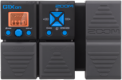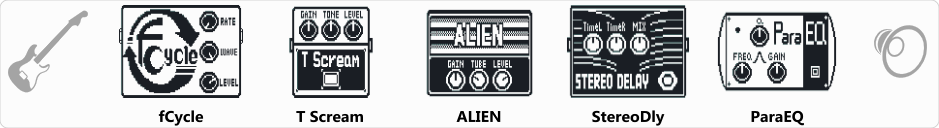Discussion in 'Zoom G1on/G1Xon' started by Kovács László, Sep 20, 2018.Device: Zoom G1Xon
Firmware: 1.21

Optimized for: Phones/Speaker

Effects chain:fCycle effekt almost like AWAH, but not picking sensitive.

Effect: "fCycle" (Dynamics / Filter), active - "yes"
"Rate" = 6
"Wave" = Sine
"Level" = 100
"Depth" = 100
"Reso" = 8

Effect: "T Scream" (Overdrive / Distortion), active - "yes"
"Gain" = 40
"Tone" = 43
"Level" = 105

Effect: "ALIEN" (Amp simulators), active - "yes"
"Gain" = 9
"Tube" = 26
"Level" = 58
"Trebl" = 34
"Middl" = 38
"Bass" = 11
"Prese" = 0
"CAB" = ALIEN 4x12
"OUT" = LINE

Effect: "StereoDly" (Delay / Reverb), active - "yes"
"TimeL" = 634
"TimeR" = 325
"Mix" = 64
"LchFB" = 29
"RchFB" = 71
"Level" = 150
"LchLv" = 79
"RchLv" = 61
"Tail" = On

Effect: "ParaEQ" (Dynamics / Filter), active - "yes"
"Freq1" = 3.2kHz
"Q1" = 0.5
"Gain1" = 10
"Freq2" = 8.0kHz
"Q2" = 1
"Gain2" = -10
"Level" = 100
Patch Volume: 110

Note: This is a patch file, you will need to download and install the ToneLib software to use the patch.